# The Lean Theorem Prover

## The Lean Theorem Prover Team

• The presenters, and
• Floris van Doorn (CMU),
• Rob Lewis (CMU),
• Daniel Selsam (Stanford)

## Many thanks to

• Soonho Kong (former member)
• Jakob von Raumer (former member)
• Cody Roux
• Georges Gonthier
• Grant Passmore
• Nikhil Swamy
• Assia Mahboubi
• Bas Spitters
• Steve Awodey
• Ulrik Buchholtz
• Tom Ball
• Parikshit Khanna
• Haitao Zhang

## Introduction: Lean

• New open source theorem prover
• Platform for
• Software verification & development
• Formalized mathematics
• Education (mathematics, logic, computer science)
• Synthesis (proofs & programs)
• de Bruijn's Principle: small trusted kernel
• Expressive logic
• Partial constructions: automation fills the "holes"
• Meta-programming

## Main Goal

Lean aims to bring two worlds together

• An interactive theorem prover with powerful automation
• An automated reasoning tool that
• produces (detailed) proofs,
• has a rich language,
• can be used interactively, and
• is built on a verified mathematical library
• A programming environment in which one can
• compute with objects with a precise formal semantics,
• reason about the results of computation, and
• write proof-producing automation
• It is an ongoing and long long term effort

## What is new?

• Meta-programming
• Extend Lean using Lean
• Access Lean internals using Lean
• Proof/Program synthesis
• Powerful elaboration engine that can handle
• Higher-order unification
• Type classes
• Coercions

"By relieving the brain of all unnecessary work, a good notation sets it free to concentrate on more advanced problems, and in effect increases the mental power of the race." – A. N. Whitehead

## What is new?

• Small trusted kernel
• It does not contain
• Termination checker
• Fixpoint operators
• Pattern matching
• Module management
• Reference type checker in Haskell
• Multi-core support
• Compile files in parallel
• Compile theorems in parallel

## What is new?

• Simple json-based protocol for communicating with editors
• Fast incremental compilation
• Auto completion
• Type information
• Goal visualization
• We already support: Emacs, VS Code and ACE editor
• Profiler and Debugger for Lean code
• We can use them to profile/debug tactics since tactics are written in Lean.

## What is new?

• Bytecode and C++ generator
• Many efficient native tactics implemented in C++
• Simplifier
• Congruence closure
• E-matching
• Ground AC completion
• (more coming soon)

## Agenda

• Lean language
• Tactics and Meta-programming
• SMT-based tactics
• Superposition prover
• Profiler and Debugger
• Native code generator

## The Logical Framework

• Dependent lambda calculus
Type -- Sort nat -- Constant λ x : nat, x -- Lambda abstraction vector bool 3 -- Application Π (n : nat), vector nat n -- Function Space nat → bool -- Function Space (no dependency)

## The Logical Framework

def f (α β : Type) : α → β → α := λ (a : α) (b : β), a def f (α β : Type) (a : α) (b : β) : α := a

## The Logical Framework

• Hierarchy of universes
check Prop -- Prop : Type 1 check Type 0 -- Prop : Type 1 check Type -- Type 1 : Type 2 check Type 1 -- Type 1 : Type 2 check Type 2 -- Type 2 : Type 3
• Universe polymorphic definitions
def {u} f (α β : Type u) : α → β → α := λ (a : α) (b : β), a universe variable u def g (α β : Type u) : α → β → α := λ (a : α) (b : β), a def h (α β : Type _) : α → β → α := λ (a : α) (b : β), a def r (α β : Type*) : α → β → α := λ (a : α) (b : β), a

## Implicit arguments

def f (α β : Type u) : α → β → α := λ (a : α) (b : β), a check f nat nat 0 1 check f _ _ 0 1 def g {α β : Type u} : α → β → α := λ (a : α) (b : β), a check g 0 1 set_option pp.implicit true check g 0 1

## The Logical Framework

• Chapter 7, Theorem Proving in Lean
• Inductive families

inductive nat | zero : nat | succ : nat → nat inductive tree (α : Type u) | leaf : α → tree | node : tree → tree → tree inductive vector (α : Type) : nat → Type | nil : vector zero | cons : Π {n : nat}, α → vector n → vector (succ n)

## Inductive families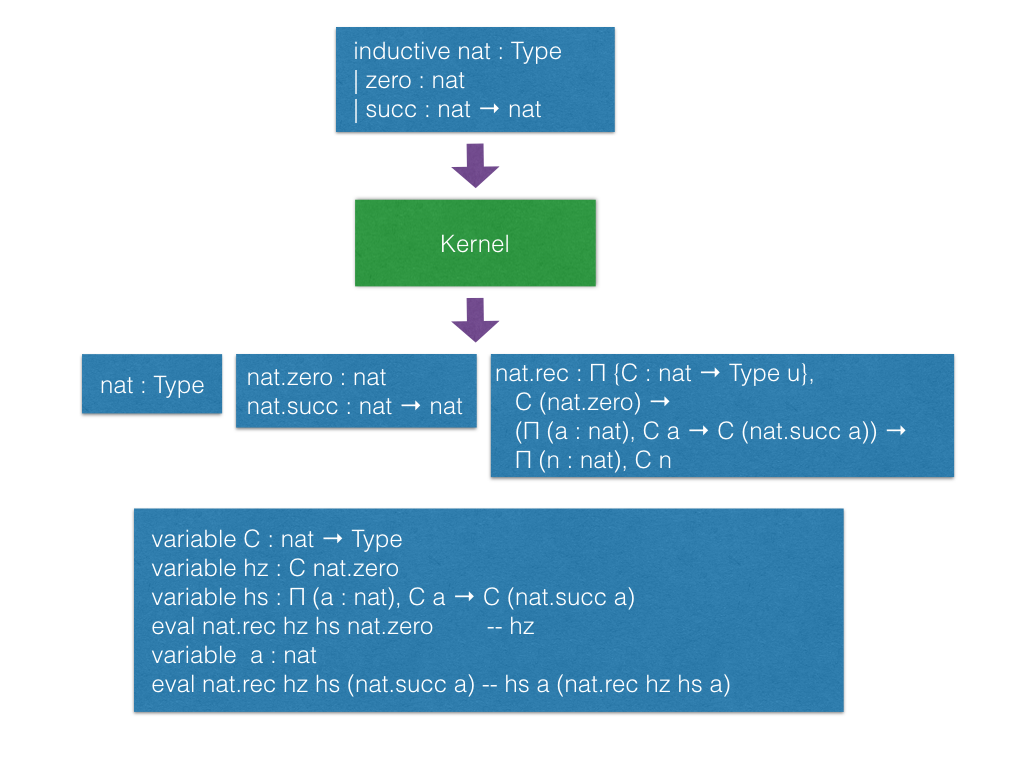## Recursive equations

• Chapter 8, Theorem Proving in Lean
• Recursors are inconvenient to use.
• Compiler from recursive equations to recursors.
• Two compilation strategies: structural and well-founded recursion
• Well-founded recursion is coming soon.
def fib : nat → nat | 0 := 1 | 1 := 1 | (a+2) := fib (a+1) + fib a example (a : nat) : fib (a+2) = fib (a+1) + fib a := rfl
• Proofs by induction

## Recursive equations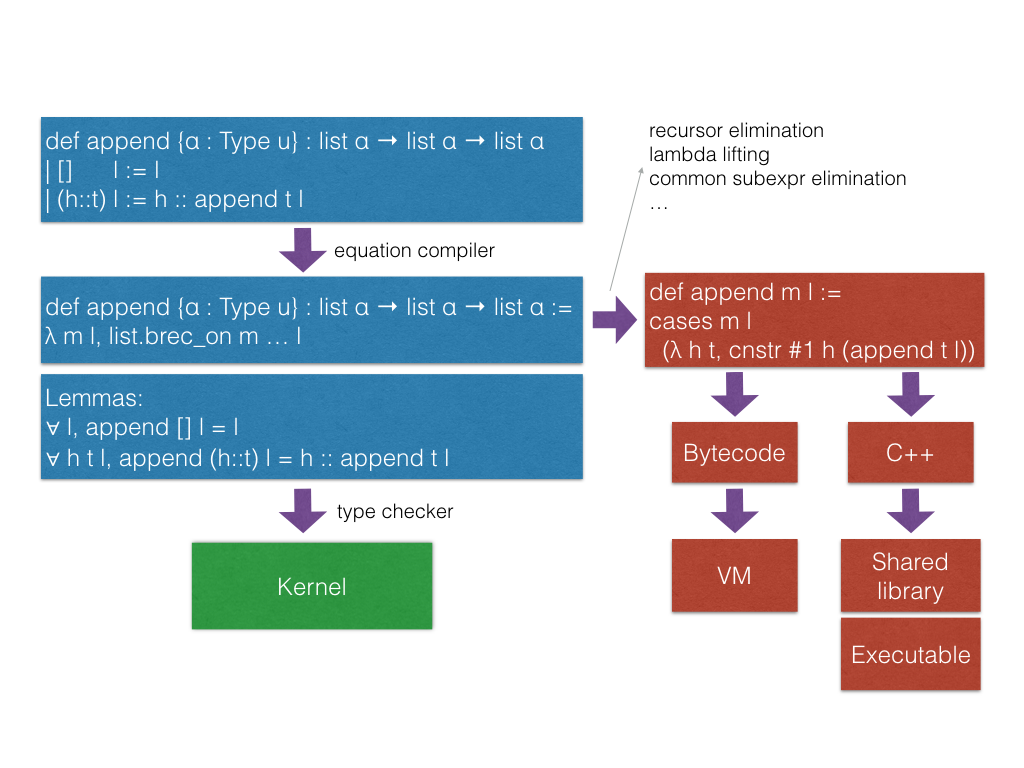## Recursive equations

• Dependent pattern matching
open nat inductive vector (α : Type) : nat → Type | nil {} : vector 0 | cons : Π {n}, α → vector n → vector (succ n) open vector def map {α β δ : Type} (f : α → β → δ) : Π {n : nat}, vector α n → vector β n → vector δ n | 0 nil nil := nil | (succ n) (cons a va) (cons b vb) := cons (f a b) (map va vb) def zip {α β : Type} : Π {n}, vector α n → vector β n → vector (α × β) n | 0 nil nil := nil | (succ n) (cons a va) (cons b vb) := cons (a, b) (zip va vb)

## Structures

structure point (α : Type) := mk :: (x : α) (y : α) eval point.x (point.mk 10 20) eval point.y (point.mk 10 20) eval {point . x := 10, y := 20} def p : point nat := {x := 10, y := 20} eval p^.x eval p^.y eval {p with x := 0} structure point3d (α : Type) extends point α := (z : α)

## Type classes

class has_sizeof (α : Type u) := (sizeof : α → nat) variables {α : Type u} {β : Type v} def sizeof [has_sizeof α] : α → nat instance : has_sizeof nat := ⟨λ a : nat, a⟩ -- ⟨...⟩ is the anonymous constructor instance [has_sizeof α] [has_sizeof β] : has_sizeof (prod α β) := ⟨λ p, match p with | (a, b) := sizeof a + sizeof b + 1 end⟩ instance [has_sizeof α] [has_sizeof β] : has_sizeof (sum α β) := ⟨λ s, match s with | inl a := sizeof a + 1 | inr b := sizeof b + 1 end⟩ vm_eval sizeof (10, 20) -- 31

## Type classes

class inhabited (α : Type u) := (default : α) class inductive decidable (p : Prop) | is_false : ¬p → decidable | is_true : p → decidable class has_one (α : Type u) := (one : α) class has_add (α : Type u) := (add : α → α → α) class has_mul (α : Type u) := (mul : α → α → α) class semigroup (α : Type) extends has_mul α := (mul_assoc : ∀ a b c : α, a * b * c = a * (b * c)) class monoid (α : Type) extends semigroup α, has_one α := (one_mul : ∀ a : α, 1 * a = a) (mul_one : ∀ a : α, a * 1 = a) class functor (f : Type u → Type v) := (map : Π {α β : Type u}, (α → β) → f α → f β) class monad (m : Type u → Type v) extends functor m := (ret : Π {α : Type u}, α → M α) (bind : Π {α β : Type u}, M α → (α → m β) → m β)

## Coercions as type classes

class has_coe (α : Type u) (β : Type v) := (coe : α → β) instance coe_bool_to_Prop : has_coe bool Prop := ⟨λ b, b = tt⟩ structure subtype {α : Type u} (p : α → Prop) := (elt_of : α) (has_property : p elt_of) instance coe_sub {α : Type u} {p : α → Prop} : has_coe (subtype p) α := ⟨λ s : subtype, elt_of s⟩ def below (n : nat) : nat → Prop := λ i, i < n def f {n : nat} (v : subtype (below n)) : nat := v + 1 --^ Coercion used here

## Meta-programming

• Extending Lean in Lean
• Lean has an efficient bytecode interpreter

inductive expr | var : unsigned → expr | sort : level → expr | const : name → list level → expr | app : expr → expr → expr ... meta constant tactic_state : Type

## Meta-programming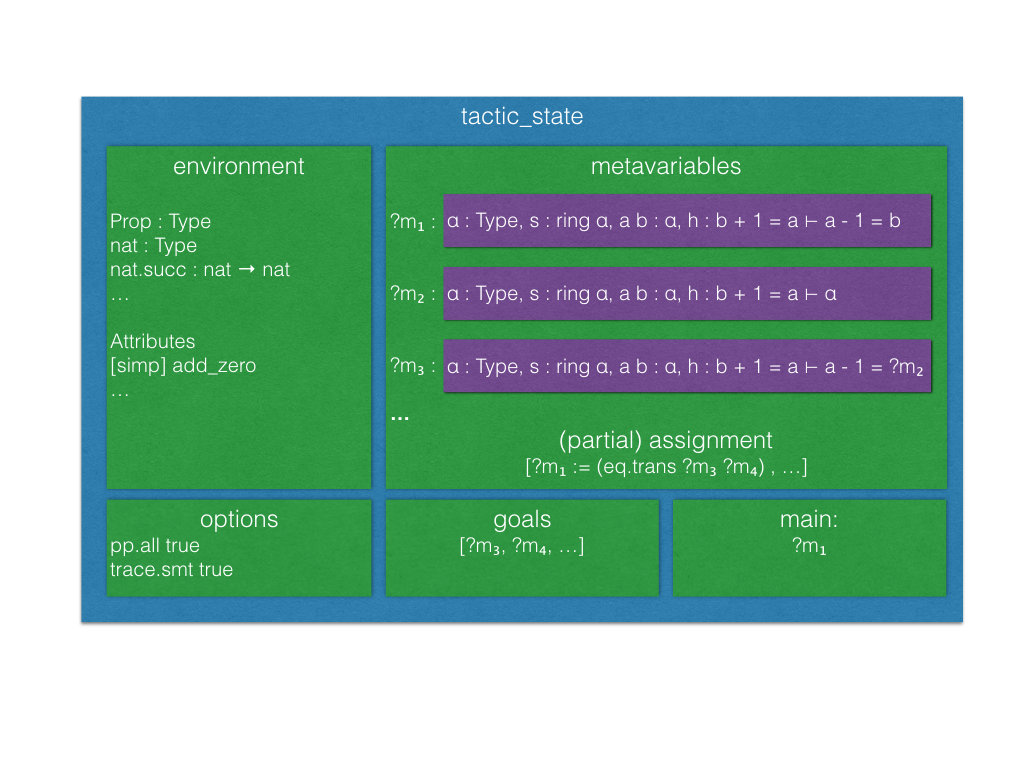## Meta-programming

inductive tactic_result (α : Type) | success : α → tactic_state → tactic_result | exception : (unit → format) → tactic_state → tactic_result meta def tactic (α : Type) := tactic_state → tactic_result α meta instance : monad tactic := ... meta constant infer_type : expr → tactic expr meta constant subst : expr → tactic unit meta constant exact : expr → tactic unit meta constant local_context : expr → tactic (list expr) meta constant to_expr : pexpr → tactic expr meta def apply : expr → tactic unit := ...

## Meta-programming

• The by tac instructs Lean to use tac : tactic unit to synthesize the missing term.
• Demo

## Interactive mode

• Many users want to apply tactics interactively.
• They want to observe intermediate tactic_state's.
• They want to avoid quotations, and gloss over the distinction between object and meta expressions.

-- They want to write exact eq.trans h₁ (eq.symm h₂) -- instead of to_expr (eq.trans h₁ (eq.symm h₂)) >>= exact -- or the following, assuming (exact : pexpr -> tactic unit) exact (eq.trans h₁ (eq.symm h₂))
• Lean provides an "interactive mode" for applying tactics.
• Demo

## SMT tactic framework

• It implements gadgets found in state-of-the-art SMT solvers
• Congruence closure
• E-matching
• Unit propagation
• AC
• Arithmetic (coming soon)
• smt_goal contains the state of the SMT "gadgets" for a particular goal.
• "Attaching more state to tactic_state".

meta constant smt_goal : Type meta def smt_state := list smt_goal meta def smt_tactic := state_t smt_state tactic
• Users can solve problems interactively, and/or write their own "end game" tactics.
• We provide Lean APIs for traversing equivalence classes, inspecting instances and lemmas used for E-matching, etc.

## Lifting tactic ==> smt_tactic

• Any tactic that does not change the set of hypotheses can be easily lifted because they do not invalidate the smt_state.
• Tactic smt_tactic.intros
• Add new hypothesis, and update smt_state.
• It will update equivalence classes, propagate equalities, etc.
• Tactics that modify the set of hypotheses (e.g., revert, induction) can be lifted, but the affected smt_goal's are reconstructed from scratch.

## SMT interactive mode

lemma app_assoc (l₁ l₂ l₃ : list α) : app (app l₁ l₂) l₃ = app l₁ (app l₂ l₃) := begin [smt] induction l₁, all_goals {eblast} end

## Superposition prover

• Implemented 100% in Lean
• 2200 lines of code
• including toy SAT solver
• Uses Lean expressions, unification, proofs
• Complete for first-order logic with equality
• Similar to metis in Isabelle

## Superposition prover

• Based on refutation of formulas in clause normal form (CNF)
p 3 → (∀x, p x → q (x+1)) → q 4 -- negated for refutation: ¬(p 3 → (∀x, p x → q (x+1)) → q 4) -- in CNF: p 3 ∧ (∀x, ¬p x ∨ q (x+1)) ∧ ¬q 4 -- super's trace output: [[p 3], [¬p ?m_1, q (?m_1 + 1)], [¬q 4]]
• Applies inferences until contradiction (empty clause)

## Superposition prover

• Inferences (modulo unification)
-- resolution [a, b] & [¬b, c] ==> [a, c] -- superposition ("rewriting with conditions") [a, t=s] & [b t, c] ==> [a, b s, c]
• and others

## Superposition prover

• Term ordering
• Selection
• Subsumption
• Demodulation
• Splitting
• "Best-effort" intuitionist proofs
• To be done:
• Term indexing
• AC redundancy elimination

## Superposition prover

• State transformer of tactic monad
meta structure prover_state := (active : rb_map clause_id derived_clause) (passive : rb_map clause_id derived_clause) (prec : list expr) (sat_solver : cdcl.state) -- ... meta def prover := state_t prover_state tactic

## Superposition prover demos

• usage.lean: shows the basic usage of the super tactic
• clauses.lean: shows the data structure used for clauses
• assoc.lean: support for associative-commutative function symbols; as an example we show how to obtain the right inverse from the left inverse in monoids
• listex.lean: reasoning about membership in lists
• heapex.lean: proving a lemma about the disjoint union of heaps in separation logic, [that we proved before using E-matching](TODO)

## Superposition prover

• Future work
• Performance
• Application of simplification rules
• Use of standard library
• Integration with SMT tactics
• Better clause representation
• Heterogeneous equality
• Configurability
• "Leanhammer"

## Profiler

• Based on sampling
• It takes snapshots of the VM stack every x ms (default: 10 ms)
• Useful for finding performance bottlenecks in tactics
• Demo

## Debugger

• We can monitor the VM execution.
meta constant get_env : vm environment meta constant stack_size : vm nat meta constant stack_obj : nat → vm vm_obj ... meta structure vm_monitor (s : Type) := (init : s) (step : s → vm s) meta constant vm_monitor.register : name → command

## Debugger

• Lean comes with a simple CLI debugger implemented in Lean on top of the VM monitor API.
namespace debugger meta def step_fn (s : state) : vm state := do s ← prune_active_bps s, ... else return s meta def monitor : vm_monitor state := { init := init_state, step := step_fn } end debugger run_command vm_monitor.register debugger.monitor

## Native Compiler

• Lean is able to compile your programs no configuration needed
import system.io def main : io unit := put_str_ln "Hello Lean!"
lean --compile hello.lean
./hello
"Hello Lean!"

• Goal is to produce efficient native code given a Lean term
• Assign computational intepretations to programs outside the logic (i.e io)
• Verify and execute programs without friction

## Native Compiler Design

• Use C++ as a high level assembler, makes code generation, linking with runtime, and calling into the VM easy
• Current compiler is the third generation
• Initial prototype was implemented in C++
• How to increase:
• Confidence in correctness
• Ease of implementation
• Reusability

## Self Hosting

• Application of a repeated theme: script Lean in Lean
• shares phases with VM compiler
• Transform higher order, dependently typed lambda calculus to ir:
• an imperative language in A Normal Form.
• Denote IR into C++
• Easier to prototype and configure then LLVM
• Easily call runtime primitives
• Rely on standard C++ optimizations
• Enables verification of the compiler from expr to ir

## Self Hosting

• A fragment of the IR compiler written in Lean
meta def compile_defn_to_ir (decl_name : name) (params : list name) (body : expr) : ir_compiler ir.defn := do body' ← compile_expr_to_ir_stmt body, let no_params := list.length params, const_obj_ref := ir.ty.ref (ir.ty.object none), param_tys := list.repeat const_obj_ref no_params, params := (list.zip params param_tys) in pure (ir.defn.mk bool.tt decl_name params (ir.ty.object none) body')
• able to use:

@[reducible] meta def ir_compiler (A : Type) := resultT (state ir_compiler_state) error A
• Higher level operations, reduces boilerplate present in C++ prototype (i.e monad.map)
• Reason and verify properties about the compiler

## Compiler Design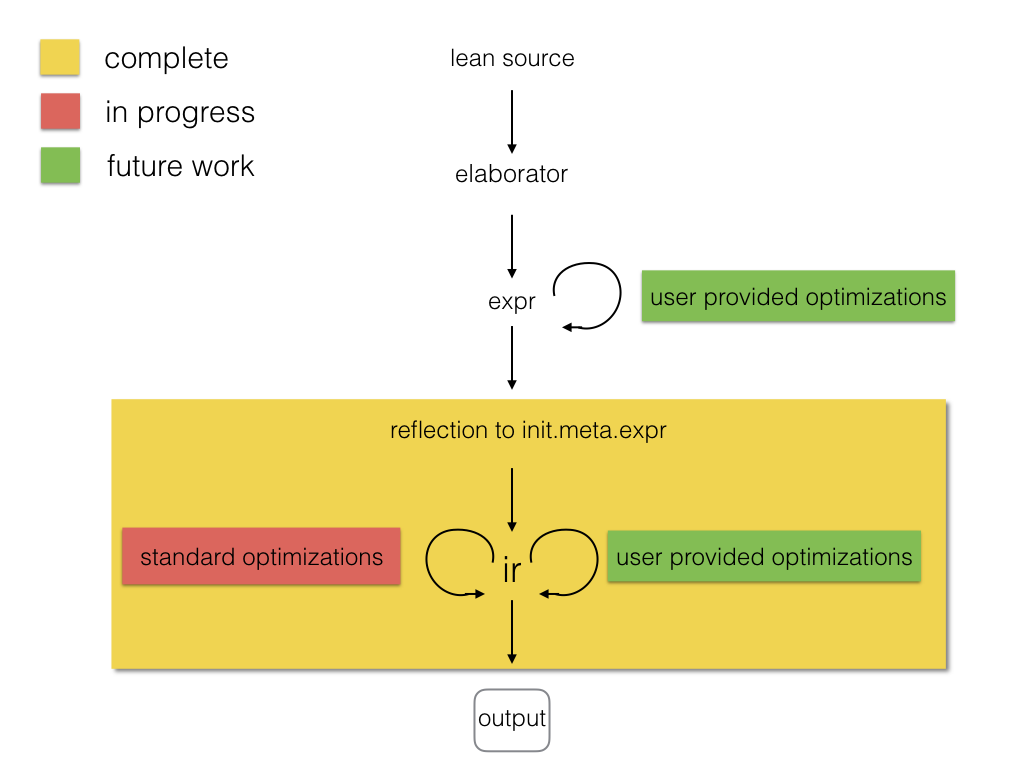## Efficient tactics

• Tactics written in Lean are accesible to average user
• Enables scripting low level automation
• Accelerate tactics using compilation, and optimization
• Build shared library per package; dynamically load at runtime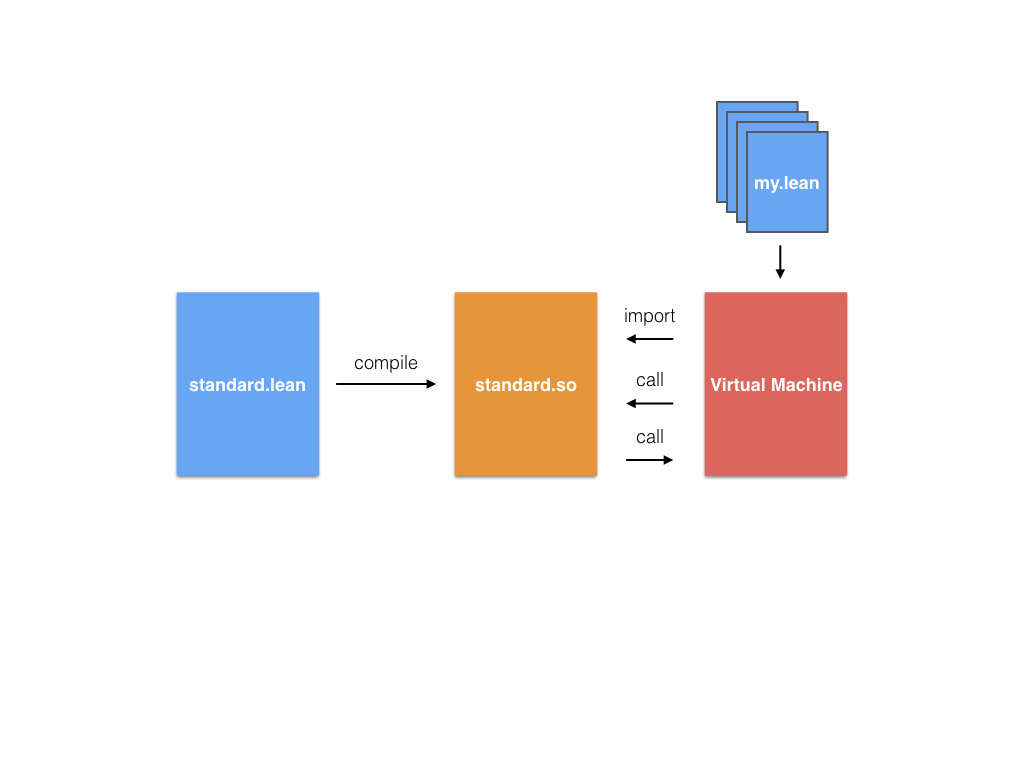## Programming

• Write a program, verify a property, compile and run
• No need to configure; convention over configuration

lean --compile my_source_code.lean

• Configuration still exists.
• Future plans for user extension, philoshophy is well-designed libraries should package:
• Inductive types and their operations
• Lemmas about types and definitions in the library
• Tactics for reasoning about the library
• Refinements for executing the library efficiently

## Work in progress

• Package level compilation
• Compile entire package into native code, which can be loaded by the VM
• Implemented but needs multi-platform support and polish
• Allow user provided IR refinements, with refinement proofs
• Basic support for replacing types + operations with implementations in IR
• The version of the native compiler in master is incomplete
• The next iteration is nearing completion, and contains many of the features discussed above.

## Work in progress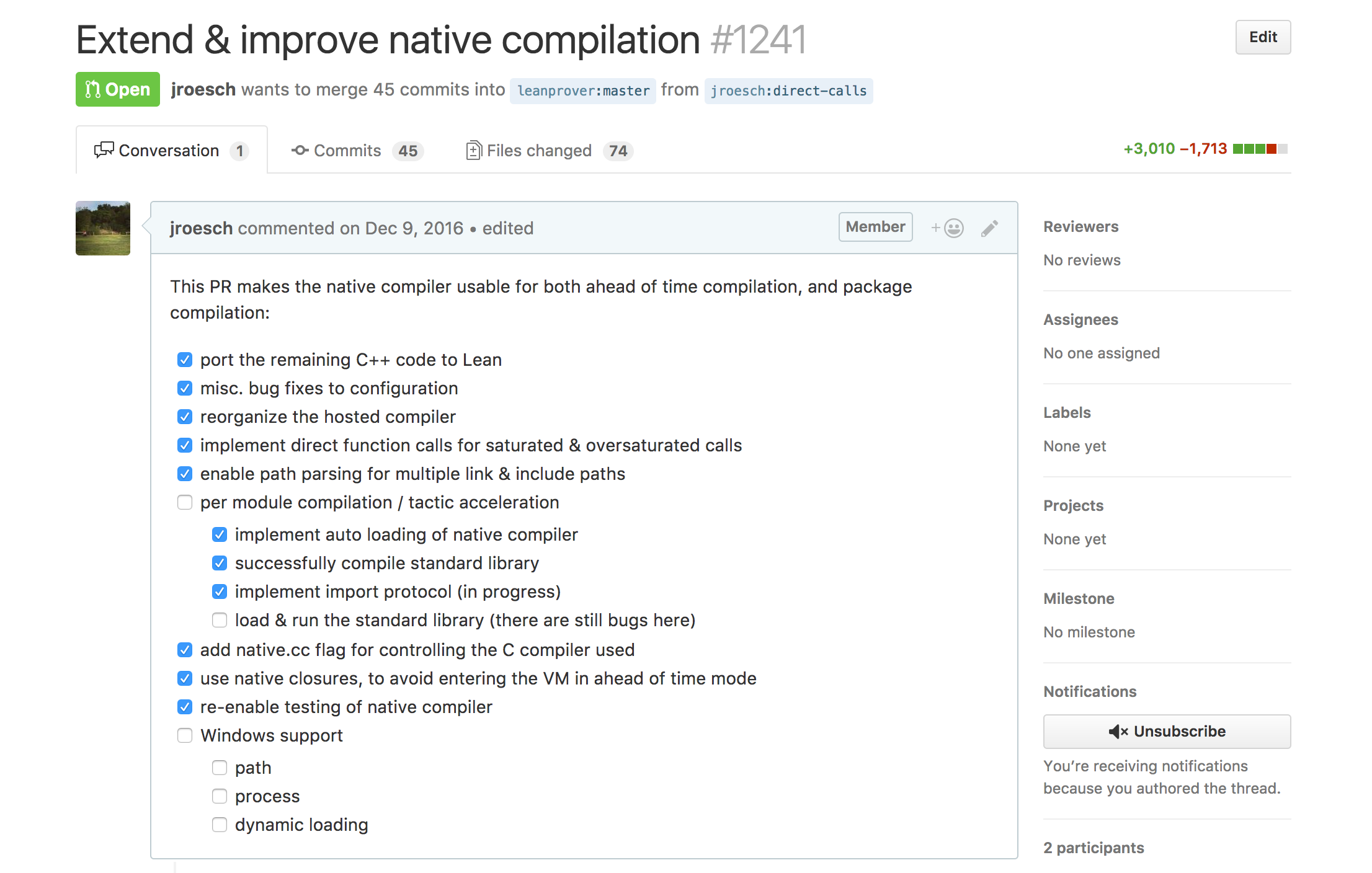## Future Work

• Framework for rewriting based on equality (in the theory, i.e eq heq)
• We can use exisiting simplifier as an optimizer
• Formally verifiy compiler
• Give formal semantics to all IRs
• Finish IR typechecker, and type system
• Apply traditional compiler verification techniques (CompCert, …)

## Native Compiler Demo

• Configuring the native compiler
• Inspecting generated code
• Executing it in ahead of time mode
• More

## Project suggestions

• Write tactics for automating your favorite project.
• Hoogle for Lean
• The Lean API provides functions/tactics for traversing the environment (environment.fold), type inference (tactic.infer_type), unification (tactic.unify), etc.
• Documentation generator. The tactic tactic.doc_string retrieves the doc string for a given declaration.
• Goal visualizer. VS Code can render complex graphics and elaborated formatting, it is built on top of Chromium. The Lean goal pretty printer can be customized, we just need to define our instance for has_to_format tactic_state`.
• Type based auto completion. The idea is to filter the list of candidates using the expected type.
• Formatting tool. Build a tool to automatically format Lean code using a consistent rule set. Consider similar tools.
• Debugger interface based on the VM monitoring API. Consider using the VS Code generic debug UI.